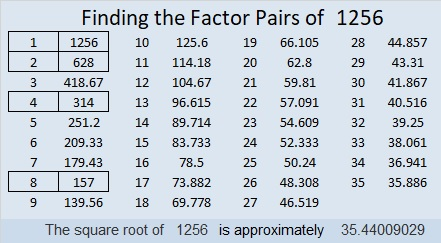# 1256 and Level 5

Use logic, not guess and check, to find where the numbers from 1 to 12 belong in both the first column and the top row so that the puzzle acts like a multiplication table. Can you do it, or will some of the clues trick you?Print the puzzles or type the solution in this excel file: 12 factors 1251-1258

Now I’ll share some facts about the number 1256:

• 1256 is a composite number.
• Prime factorization: 1256 = 2 × 2 × 2 × 157, which can be written 1256 = 2³ × 157
• The exponents in the prime factorization are 3 and 1. Adding one to each and multiplying we get (3 + 1)(1 + 1) = 4 × 2 = 8. Therefore 1256 has exactly 8 factors.
• Factors of 1256: 1, 2, 4, 8, 157, 314, 628, 1256
• Factor pairs: 1256 = 1 × 1256, 2 × 628, 4 × 314, or 8 × 157
• Taking the factor pair with the largest square number factor, we get √1256 = (√4)(√314) = 2√314 ≈ 35.440091256 is the sum of two squares:
34² + 10² = 1256

1256 is the hypotenuse of a Pythagorean triple:
680-1056-1256 which is 8 times (85-132-157) and
can also be calculated from 2(34)(10), 34² – 10², 34² + 10²

1256 is 888 in BASE 12 because 8(144 + 12 + 1) = 8(157) = 1256

This site uses Akismet to reduce spam. Learn how your comment data is processed.